The 45 Degree Rule of Thumb for Surcharges

18 Apr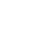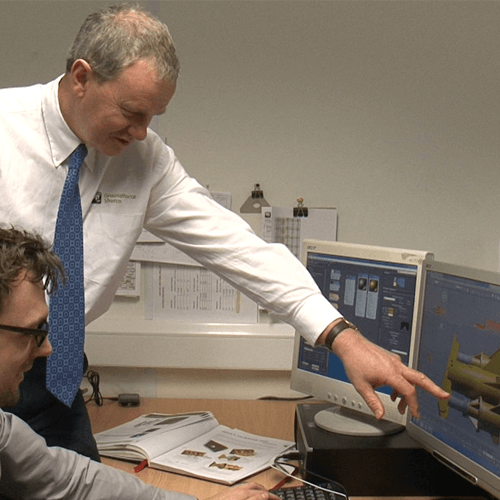This blog returns to the more technical theme of temporary works design and poses the question:

Why do we so often apply the 45° rule of thumb for the influence that surcharges have on the design of excavation support?

The various equations we use to calculate the effect of surcharges demonstrate that there is still an effect (albeit a small one) far beyond this arbitrary 45° zone. Why, then, do we stick to this figure for assessing if and when a surcharge will adversely affect the lateral earth pressure on retaining structures? Is this figure conservative enough? Or perhaps it is too conservative.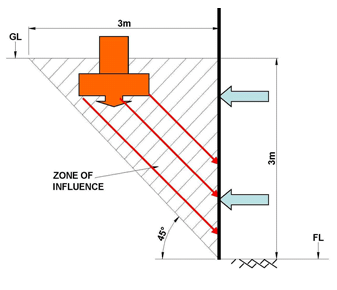Discussion

There are many reasons why we might use 45°, some of which relate to the security of the nearby structure rather than to earth pressure. For now we will just take a look from a temporary works perspective and assess what effect a surcharge will have in terms of additional loads on the shoring that supports the wall.

So, let’s keep things simple for now and just consider a line load surcharge of, say, 10kN/m acting at the surface close to the excavation. According to classic soil mechanics theory, we can assess the lateral pressure developed from this surcharge by using a modified version of Boussinesq’s elastic bearing pressure equations. Note there are many other ways of assessing surcharge effects but let us stick with this for now.

This modified version looks a little like this, where Instantaneous lateral pressure (px) at depth (NH) is represented by the equation: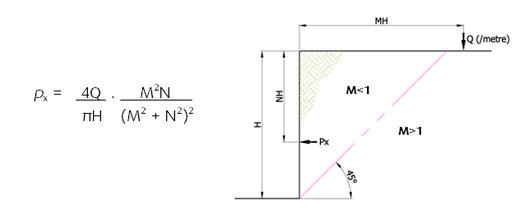Those who are lucky to be familiar with Boussinesq’s equations may recognise the unmodified version of the equation as: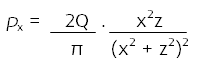Notice that the coefficient of Q in this case is 2 and not 4. The reason for doubling the coefficient of Q in our modified equation is explained quite well by Craig’s soil Mechanics: “the structure will tend to interfere with horizontal strain… a second load Q must be imagined at an equal distance on the other side…”. This takes a small mathematical leap of faith, but essentially: the soil wants to move and in order to stop it; the wall must push back with an equal and opposite force, hence doubling the effective lateral pressure.

At this point it’s important to note that Boussinesq’s equations are for elastic, homogeneous, isotropic (in otherwords, uniform) material. Consequently these equations don’t take into account the soil parameters of the material being retained.

Applying the modified Boussinesq equation 1: The graph below shows the pressure distribution on an H =1m deep retaining structure caused by our notional 10kN/m line load surcharge (Q) as we vary the distance of the surcharge from the back of the wall (M).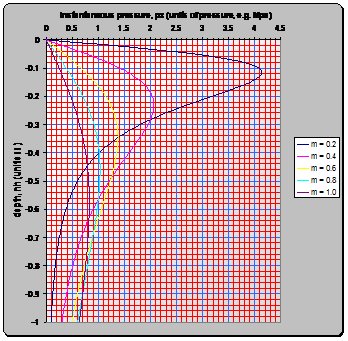Obviously, the 45 degree rule of thumb line occurs when M=1, i.e. when the distance from the excavation is equal to the depth of the excavation. You will note that the pressure increases significantly as the surcharge gets nearer the wall (again fairly obviously); which is why we need to account for surcharges within our 45° “zone of influence”; and the surcharge will have much less effect as M exceeds 1, when the surcharge lies outside the 45° “zone of influence”. Purists will argue that surcharges need to be allowed for even when they lie outside the zone of influence; and this is certainly a necessity for permanent works designs. By contrast most temporary works engineers will work to the 45° rule, at least for relatively low risk excavations. You can see from the graph here that as M increases, not only does the pressure decrease as stated earlier, but the concentration of this pressure shifts lower and lower. It is easy to imagine that at a certain point, the surcharge will be supported by the earth that lies under the excavation and will no longer have a significant effect on the shoring system.

To finish off the brain ache session we can calculate the magnitude of the load acting on the back of the wall; which is what we are looking for after all. We can easily convert our modified Boussinesq equation from instantaneous pressure to total lateral load by performing an integration with respect to N for N=0 to N=1; in other words, calculate the area to the left of the line in the graph above. For those of you interested in equations, this is represented by:-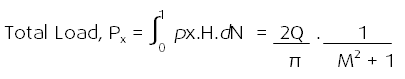What is interesting to note here is that this expression is now independent of the H and N variables. Therefore it is independent of the depth of the excavation.

This can be plotted as per the graph below to show the magnitude of total lateral load generated from the same 10kN/m line load surcharge acting at different distances from the excavation. Since this expression is independent of depth, these values are true for any depth of excavation.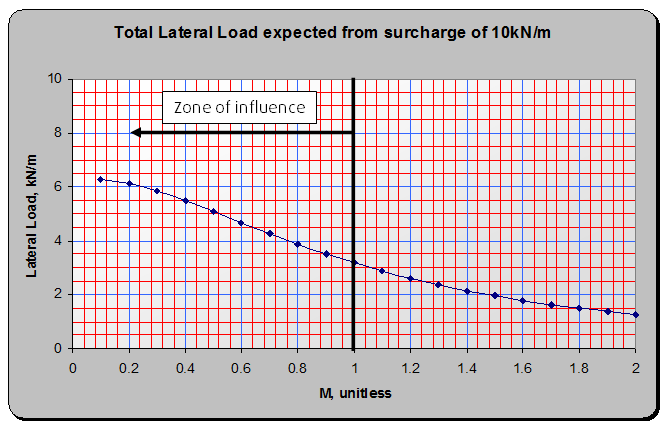These values essentially represent the additional line load being applied to a suitably positioned bracing frame due to the surcharge.

To finish off let us look at two examples of the 10kN/m line surcharge acting at just past the 45° threshold:

In this example, the surcharge from a 10kN/m line load located just outside the area of influence could contribute and extra 113% to the load on the shoring frame.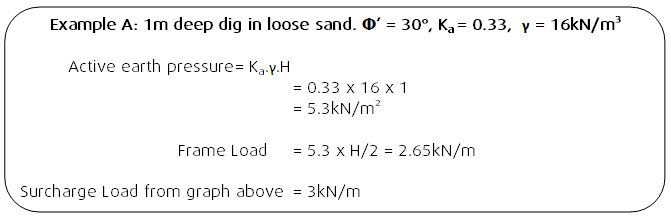In this example, even thought the surcharge load is the same, relative to the size of the earth pressures, it seems much less significant.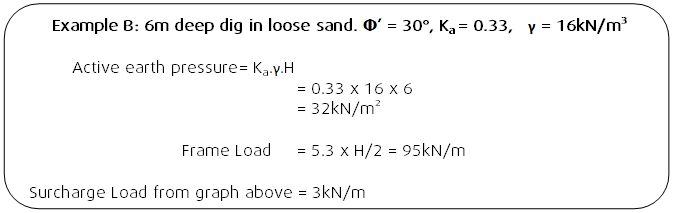Summary

The theories set out above give an indication for why temporary works engineers adopt the 45 degree rule of thumb. What is clear is that beyond 45°, the size of the load has reduced in magnitude and the effective centre of the load has moved deeper. The only discrepancy this leaves is in very shallow excavations with very close surcharges. In these cases, a little rational thought and engineering judgement should be used.

It is up to individual engineer to decide whether or not to apply this rule to their detailed calculations and as ever it should be assessed on a job by job basis. Other rules of thumb are adopted by various sectors. In the rail sector, for instance, it is common to see a 45° line offset 3m back from the retaining wall. This certainly solves the discrepancy seen in example A above.

The 45° rule of thumb remains, however, a very convenient method of performing a speedy on-site assessment of surcharges; and in the hands of competent people has remained a robust tool for many years.

Tony Gould